## How to make multiple selections in a SAS VIYA list lead to multiple lines in a time series plot

I'm trying to make a time series plot based on a table with 5 columns: three character columns which determine where the data comes from and what it's about, the date the data was collected, and the value of some variable at that time.

I have three lists, one for each character column, which allow the user to choose which data to look at in a time series plot, as shown below.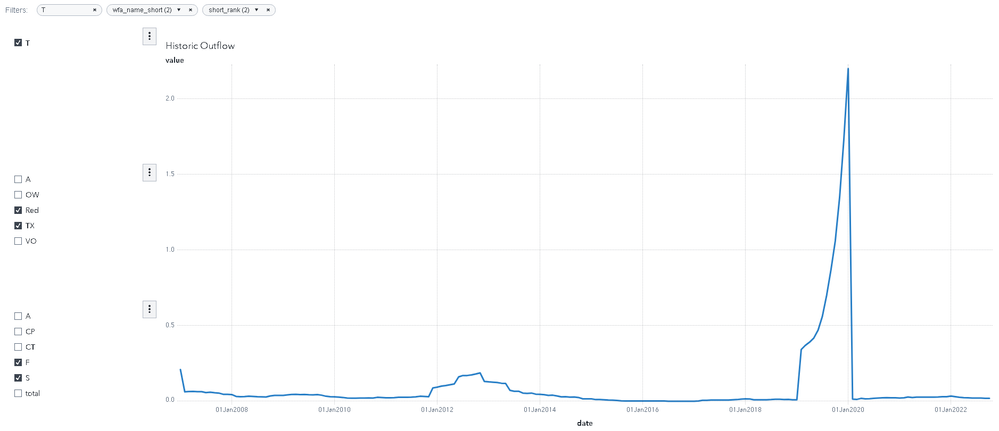What I have

When the user selects multiple items in the lists, SAS adds all of the values that satisfy the filter and displays the total in a single line.

What I want is for SAS to create one line for each possible combination of ticked items in the list to allow them to look at multiple sources of data at the same time. In this case, as there is 1 item selected in the first character column, 2 in the second and 2 in the third, I would like 1x2x2=4 lines, as shown below.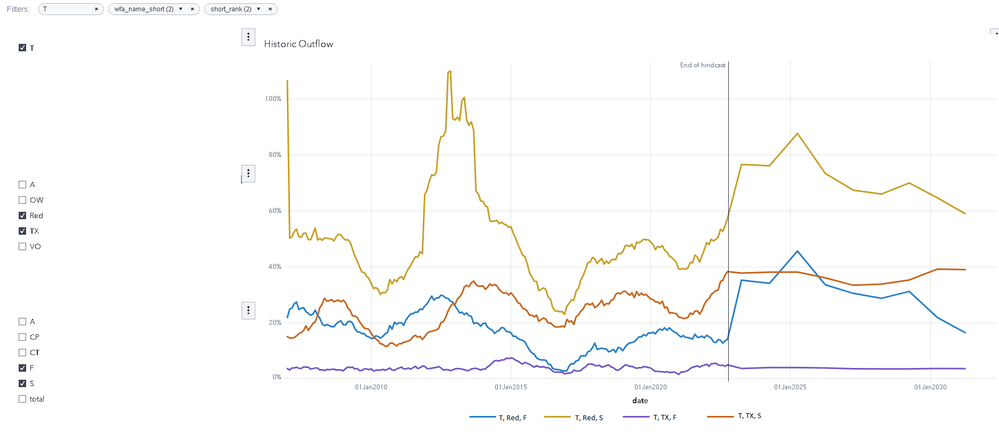What I want, made in MS Paint

I made the above image using MS Paint and an unrelated time series plot that uses multiple Measures, which is why the data is inconsistent.

So far, my best attempt at getting a multi-line time series plot was by transposing the table on the three character columns using PROC TRANSPOSE, uploading the modified dataset to CAS, then using the columns representing each combination of data sources in the "Measure" parameter of the time series plot.

Unfortunately, this doesn't allow the user to choose which columns are shown without access to editing mode, as I don't know how to get the user to choose which columns are put in the "Measure" parameter of a time series plot without editing the plot itself. If there is a way to let the user choose which columns to analyse in a time series plot, that would be an acceptable (albeit suboptimal) solution.

If you know SAS VIYA can't do this (or I need to use something other than a time series plot), please tell me so I can seek an alternative solution.

1 ACCEPTED SOLUTION

Accepted Solutions

## Re: How to make multiple selections in a SAS VIYA list lead to multiple lines in a time series plot

Without knowing what your data looks like here is a possible solution with fake data.

The data for the example looks like this, as you can see I have two category columns, a date column and a value column.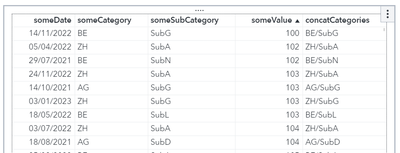Steps:

• Create a new graph object using "Custom Graph Builder". The custom graph contains a Time Series plot. This plot has two roles by default, add a third role of type Group.
• Import the new custom graph into your object list.
• Create a report page consisting of two List controls and the new custom graph timeseries plot
• The two List controls take their values from someCategory and someSubCategory, I made both required
• Assign each List control a parameter that supports multiple values
• Create a new calculated Data Item using the Concatenate operator like so:
Concatenate(Concatenate('someCategory'n, '/'), 'someSubCategory'n)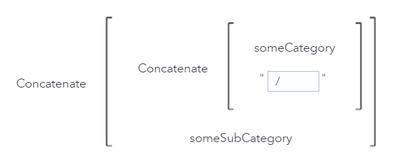• On the custom graph time series plot assign the proper roles, someDate for the X axis, someValue for the Y axis and the new concatCategories created above as the group
• On the custom graph time series plot add an advanced filter that will filter based on the selected values from the two List controls (the values are in the parameters), the filter looks like this (where the names to the right on the In operator are the parameter names):The resulting report will look like this: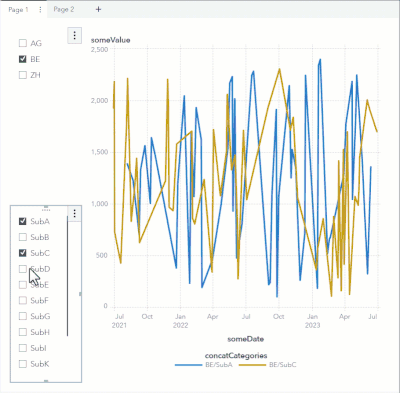Give it a try

## Re: How to make multiple selections in a SAS VIYA list lead to multiple lines in a time series plot

Without knowing what your data looks like here is a possible solution with fake data.

The data for the example looks like this, as you can see I have two category columns, a date column and a value column.Steps:

• Create a new graph object using "Custom Graph Builder". The custom graph contains a Time Series plot. This plot has two roles by default, add a third role of type Group.
• Import the new custom graph into your object list.
• Create a report page consisting of two List controls and the new custom graph timeseries plot
• The two List controls take their values from someCategory and someSubCategory, I made both required
• Assign each List control a parameter that supports multiple values
• Create a new calculated Data Item using the Concatenate operator like so:
Concatenate(Concatenate('someCategory'n, '/'), 'someSubCategory'n)• On the custom graph time series plot assign the proper roles, someDate for the X axis, someValue for the Y axis and the new concatCategories created above as the group
• On the custom graph time series plot add an advanced filter that will filter based on the selected values from the two List controls (the values are in the parameters), the filter looks like this (where the names to the right on the In operator are the parameter names):The resulting report will look like this:Give it a try

Discussion stats